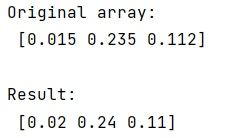# How to round a numpy array?

Learn, how to round a numpy array in Python?
Submitted by Pranit Sharma, on January 16, 2023

NumPy is an abbreviated form of Numerical Python. It is used for different types of scientific operations in python. Numpy is a vast library in python which is used for almost every kind of scientific or mathematical operation. It is itself an array which is a collection of various methods and functions for processing the arrays.

## Rounding a numpy array

Suppose that we are given a NumPy array that contains some float values, and we need to round each element up to two decimal places.

Numpy provides a method to do this. We will use numpy.ndarray.round() method for this purpose.

This method returns an array with each element rounded to the given number of decimals.

Syntax:

```ndarray.round(decimals=0, out=None)
```

Parameter(s):

• a: array_like: Input data.
• decimals: int, optional: Number of decimal places to round to (default: 0). If decimals are negative, it specifies the number of positions to the left of the decimal point.
• out: ndarray, optional: Alternative output array in which to place the result. It must have the same shape as the expected output, but the type of output values will be cast if necessary. See Output type determination for more details.

Let's understand with the help of an example,

## Python code to round a numpy array

```# Import numpy
import numpy as np

# Import pandas
import pandas as pd

# Creating a numpy array
arr = np.array([0.015, 0.235, 0.112])

# Display original array
print("Original array:\n",arr,"\n")

# Using round function
res = np.round(arr, 2)

# Display result
print("Result:\n",res)
```

Output:What's New (MCQs)

Top Interview Coding Problems/Challenges!

IncludeHelp's Blogs

Languages: » C » C++ » C++ STL » Java » Data Structure » C#.Net » Android » Kotlin » SQL
Web Technologies: » PHP » Python » JavaScript » CSS » Ajax » Node.js » Web programming/HTML
Solved programs: » C » C++ » DS » Java » C#
Aptitude que. & ans.: » C » C++ » Java » DBMS
Interview que. & ans.: » C » Embedded C » Java » SEO » HR
CS Subjects: » CS Basics » O.S. » Networks » DBMS » Embedded Systems » Cloud Computing
» Machine learning » CS Organizations » Linux » DOS
More: » Articles » Puzzles » News/Updates